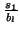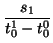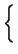Subsections

# Packet Pair Technique

In this section we describe the packet pair property of FIFO-queueing networks and show how it can be used to measure bottleneck link bandwidth.

## Packet Pair Property of FIFO-Queueing networksThe packet pair property of FIFO-queueing networks predicts the difference in arrival times of two packets of the same size traveling from the same source to the same destination:

 t1n - t0n = max, t10 - t00(1)

where t0n and t1n are the arrival times of the first and second packets respectively at the destination, t00 and t10 are the transmission times of the first and second packets respectively, s1 is the size of the second packet, and bl is the bandwidth of the bottleneck link.

The intuitive rationale for this equation (a full proof is given in [LB00]) is that if two packets are sent close enough together in time to cause the packets to queue together at the bottleneck link (> t10 - t00), then the packets will arrive at the destination with the same spacing ( t1n - t0n) as when they exited the bottleneck link (). The spacing will remain the same because the packets are the same size and no link downstream of the bottleneck link has a lower bandwidth than the bottleneck link (as shown in Figure 1, which is a variation of a figure from [Jac88]).

This property makes several assumptions that may not hold in practice. First, it assumes that the two packets queue together at the bottleneck link and at no later link. This could by violated by other packets queueing between the two packets at the bottleneck link, or packets queueing in front of the first, the second or both packets downstream of the bottleneck link. If any of these events occur, then Equation 1 does not hold. In Section 2.2, we describe how to mitigate this limitation by filtering out samples that suffer undesirable queueing.

In addition, the packet pair property assumes that the two packets are sent close enough in time that they queue together at the bottleneck link. This is a problem for very high bandwidth bottleneck links and/or for passive measurement. For example, from Equation 1, to cause queueing between two 1500 byte packets at a 1Gb/s bottleneck link, they would have to be transmitted no more than 12 microseconds apart. An active technique is more likely than a passive one to satisfy this assumption because it can control the size and transmission times of its packets. However, in Section 2.2, we describe how passive techniques can detect this problem and sometimes filter out its effect.

Another assumption of the packet pair property is that the bottleneck router uses FIFO-queueing. If the router uses fair queueing, then packet pair measures the available bandwidth of the bottleneck link [Kes91].

Finally, the packet pair property assumes that transmission delay is proportional to packet size and that routers are store-and-forward. The assumption that transmission delay is proportional to packet size may not be true if, for example, a router manages its buffers in such a way that a 128 byte packet is copied more than proportionally faster than a 129 byte packet. However, this effect is usually small enough to be ignored. The assumption that routers are store-and-forward (they receive the last bit of the packet before forwarding the first bit) is almost always true in the Internet.

Using the packet pair property, we can solve Equation 1 for bl, the bandwidth of the bottleneck link:

 bl =(2)

We call this the received bandwidth because it is bandwidth measured at the receiver. When filtering in the next section, we will also use the the bandwidth measured at the sender (the sent bandwidth):(3)

## Filtering Techniques

In this section, we describe in more detail how the assumptions in Section 2.1 can be violated in practice and how we can filter out this effect. Using measurements of the sizes and transmission and arrival times of several packets and Equation 1, we can get samples of the received bandwidth. The goal of a filtering technique is to determine which of these samples indicate the bottleneck link bandwidth and which do not. Our approach is to develop a filtering function that gives higher priority to the good samples and lower priority to the bad samples.Before describing our filtering functions, we differentiate between the kinds of samples we want to keep and those we want to filter out. Figure 2 shows one case that satisfies the assumptions of the packet pair property and three cases that do not. There are other possible scenarios but they are combinations of these cases.

Case A shows the ideal packet pair case: the packets are sent sufficiently quickly to queue at the bottleneck link and there is no queueing after the bottleneck link. In this case the bottleneck bandwidth is equal to the received bandwidth and we do not need to do any filtering.

In case B, one or more packets queue between the first and second packets, causing the second packet to fall farther behind than would have been caused by the bottleneck link. In this case, the received bandwidth is less than the bottleneck bandwidth by some unknown amount, so we should filter this sample out.

In case C, one or more packets queue before the first packet after the bottleneck link, causing the second packet to follow the first packet closer than would have been caused by the bottleneck link. In this case, the received bandwidth is greater than the bottleneck bandwidth by some unknown amount, so we should filter this sample out.

In case D, the sender does not send the two packets close enough together, so they do not queue at the bottleneck link. In this case, the received bandwidth is less than the bottleneck bandwidth by some unknown amount, so we should filter this sample out. Active techniques can avoid case D samples by sending large packets with little spacing between them, but passive techniques are susceptible to them. Examples of case D traffic are TCP acknowledgements, voice over IP traffic, remote terminal protocols like telnet and ssh, and instant messaging protocols.

### Filtering using Density EstimationTo filter out the effect of case B and C, we use the insight that samples influenced by cross traffic will tend not to correlate with each other while the case A samples will correlate strongly with each other [Pax97] [CC96b]. This is because we assume that cross traffic will have random packet sizes and will arrive randomly at the links along the path. In addition, we use the insight that packets sent with a low bandwidth that arrive with a high bandwidth are definitely from case C and can be filtered out [Pax97]. Figure 3 shows a hypothetical example of how we apply these insights. Using the second insight, we eliminate the case C samples above the received bandwidth = sent bandwidth (x = y) line. Of the remaining samples, we calculate their smoothed distribution and pick the point with the highest density as the bandwidth.

There are many ways to compute the density function of a set of samples [Pax97] [CC96b], including using a histogram. However, histograms have the disadvantages of fixed bin widths, fixed bin alignment, and uniform weighting of points within a bin. Fixed bin widths make it difficult to choose an appropriate bin width without previously knowing something about the distribution. For example, if all the samples are around 100,000, it would not be meaningful to choose a bin width of 1,000,000. On the other hand, if all the samples are around 100,000,000, a bin width of 10,000 could flatten an interesting maxima. Another disadvantage is fixed bin alignment. For example, two points could lie very close to each other on either side of a bin boundary and the bin boundary ignores that relationship. Finally, uniform weighting of points within a bin means that points close together will have the same density as points that are at opposite ends of a bin. The advantage of a histogram is its speed in computing results, but we are more interested in accuracy and robustness than in saving CPU cycles.

To avoid these problems, we use kernel density estimation [Sco92]. The idea is to define a kernel function K(t) with the propertyK(t)dt = 1 (4)

Then the density at a received bandwidth sample x is

 d (x) =K(5)

where c is the kernel width ratio, n is the number of points within c*x of x, and xi is the ith such point. We use the kernel width ratio to control the smoothness of the density function. Larger values of c give a more accurate result, but are also more computationally expensive. We use a c of 0.10. The kernel function we use is

 K(t) =(6)

This function gives greater weight to samples close to the point at which we want to estimate density, and it is simple and fast to compute.

### Filtering using the Received/Sent Bandwidth RatioAlthough density estimation is the best indicator in many situations, many case D samples can fool density estimation. For example, a host could transfer data in two directions over two different TCP connections to the same correspondent host. If data mainly flows in the forward direction, then the reverse direction would consist of many TCP acknowledgements sent with large spacings and a few data packets sent with small spacings. Figure 4 shows a possible graph of the resulting measurement samples. Density estimation would indicate a bandwidth lower than the correct one because there are so many case D samples resulting from the widely spaced acks.

We can improve the accuracy of our results if we favor samples that show evidence of actually causing queueing at the bottleneck link [LB99]. The case D samples are unlikely to have caused queueing at the bottleneck link because they are so close to the line x = y. On the other hand, the case A samples are on average far from the x = y line, meaning that they were sent with a high bandwidth but received with a lower bandwidth. This suggests that they did queue at the bottleneck link.

Using this insight we define the received/sent bandwidth ratio of a received bandwidth sample x to be

 p(x) = 1 -(7)

where s(x) is the sent bandwidth of x. We take the log of the bandwidths because bandwidths frequently differ by orders of magnitude.

Unfortunately, given two samples with the same sent bandwidth (7) favors the one with the smaller received bandwith. To counteract this, we define the received bandwidth ratio to be

 r(x) =(8)

### Composing Filtering Algorithms

We can compose the filtering algorithms described in the previous sections by normalizing their values and taking their linear combination:

 f (x) = 0.4*+ 0.3*p(x) + 0.3*r(x) (9)

where d (x)max is the maximum kernel density value. By choosing the maximum value of f (x) as the bottleneck link bandwidth, we can take into account both the density and the received/sent bandwidth ratio without favoring smaller values of x. The weighting of each of the components is arbitrary. Although it is unlikely that this is the optimal weighting, the results in Section 4.2 indicate that these weightings work well.

## Sample Window

In addition to using a filtering function, we also only use the last w bandwidth samples. This allows us to quickly detect changes in bottleneck link bandwidth (agility) while being resistant to cross traffic (stability). A large w is more stable than a small w because it will include periods without cross traffic and different sources of cross traffic that are unlikely to correlate with a particular received bandwidth. However, a large w will be less agile than a small w for essentially the same reason. It is not clear to us how to define a w for all situations, so we currently punt on the problem by making it a user-defined parameter in nettimer.

Kevin Lai 2001-01-29